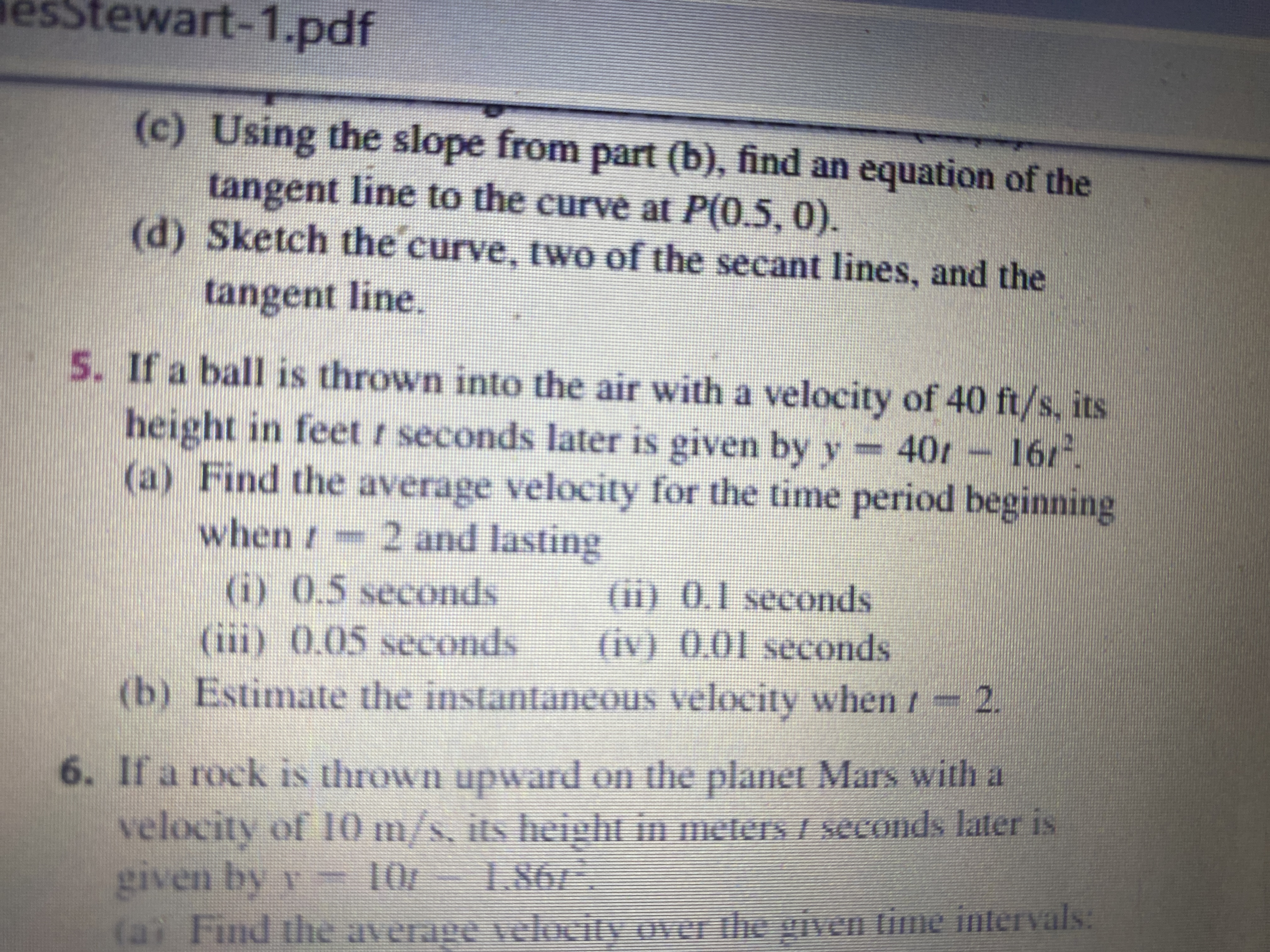# stewart-1.pdf(c) Using the slope from part (b), find an equation of thetangent line to the curve at P(0.5, 0).(d) Sketch the curve, two of the secant lines, and thetangent line.5. If a ball is thrown into the air with a velocity of 40 ft/s, itsheight in feet / seconds later is given by y16/(a) Find the average velocity for the time period beginning402 and lastingwhen /(i) 0.5 seconds(iii) 0.05 seconds(ii) 0.1 seconds(iv) 0.01 seconds2.(b) Estimate the instantaneous velocity when6. If a rock is thrown upward on the planet Mars with avelocity of 10m/s, its height in meters / seconds later isgiven by y1.8610/velocity over the given time inteIvals:(ai Find he averag

Question
2 viewshelp_outlineImage Transcriptionclosestewart-1.pdf (c) Using the slope from part (b), find an equation of the tangent line to the curve at P(0.5, 0). (d) Sketch the curve, two of the secant lines, and the tangent line. 5. If a ball is thrown into the air with a velocity of 40 ft/s, its height in feet / seconds later is given by y 16/ (a) Find the average velocity for the time period beginning 40 2 and lasting when / (i) 0.5 seconds (iii) 0.05 seconds (ii) 0.1 seconds (iv) 0.01 seconds 2. (b) Estimate the instantaneous velocity when 6. If a rock is thrown upward on the planet Mars with a velocity of 10m/s, its height in meters / seconds later is given by y 1.86 10/ velocity over the given time inteIvals: (ai Find he averag fullscreen
check_circle

Step 1

The average velocity for the time period from t0 to t1 is given by:

Step 2

(i) Find the average velocity for the time period from t0 = 2 seconds to t1 = 2 + 0.5 seconds.

Step 3

(ii) Find the average velocity for the time period ...

### Want to see the full answer?

See Solution

#### Want to see this answer and more?

Solutions are written by subject experts who are available 24/7. Questions are typically answered within 1 hour.*

See Solution
*Response times may vary by subject and question.
Tagged in

### Other